# When Does a Difference Matter? Using ANOVA to Tell

By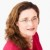Lisa Custer

Much of the Six Sigma DMAIC methodology is concerned with finding differences: Do people do a certain job the same way or are there differences? Will a particular change make a difference in the output? Are there differences in where and when a problem occurs?

In most cases, the answer to all these questions is yes. People will do things differently. Process changes will affect output. A problem will appear in some places and not others.

That is why the more important question is often “does the difference really matter?” (Or, as statisticians would say, “Are the differences significant?”) When trying to compare results across different processes, sites, operators, etc., an hypothesis testing tool that can be used to help answer that question is the analysis of variance (ANOVA).

While the theory behind ANOVA can get complicated, the good news for Six Sigma practitioners with little experience is that most of the analysis is done automatically by statistical software, so no one has to crunch a lot of numbers. Better still, the software usually produces a simple chart that visually depicts the degree of difference between items being compared – making it easy to interpret and explain to others.

A simple case study shows ANOVA in action.

### The Question: Which Site Is Fastest?

 Table 1: Collected Data Site A Site B Site C Time in minutes to complete five loan applications 15 28 26 17 25 23 18 24 20 19 27 17 24 25 21

In order to optimize the loan application process across three branches, a company wants to know which of the three locations handles the process the most efficiently. Once it determines which site is consistently fastest, the company plans to study what that site does, and adapt what it learns to the other sites. In the adjacent table is a sample of the data collected. (In real life, it is likely that more than five data points per location would be collected, but this is a simple example to illustrate the principles.)

A quick glance at this data would probably lead to the conclusion that Site B is considerably slower than Site A. (The differences are usually much harder to detect when there are a lot more data points.) But is it different from Site C? And are A and C really different?

### The ANOVA Analysis

To understand the calculations performed in an ANOVA test, a person would need to study up on statistical topics like “degrees of freedom” and “sum of squares.” Fortunately, to interpret the results, a person only needs to understand three basic concepts:

1. Mean: The mathematical average of a set of values.
2. Standard deviation: A value that represents a typical amount of variation in a set of data. (“Sigma” is the statistical notation used to represent one standard deviation; the term “Six Sigma” is used to indicated that a process is so good that six standard deviations – three above and three below the mean – fit within the specification limits)
3. p-value: A term used in hypothesis testing to indicate how likely it is that the items being compared are the same. A low p-value – often anything below 0.05 – indicates that it is very unlikely the items are the same. (Or, as non-statisticians would say, “They are different.”)
Handpicked Content:   Using ANOVA to Find Differences in Population Means

The output from the statistical software is in two parts. Figure 1 shows the first portion: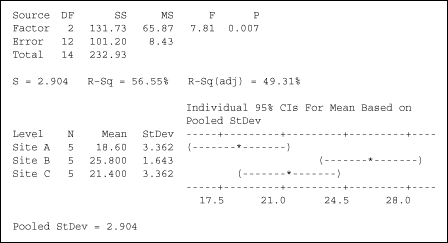Figure 1: Numerical Output – One-Way ANOVA

As can be seen, the p-value here is .007, a very small value. That shows that all three sites are not the same, but it does not indicate in what ways they differ. For that, the second part of the ANOVA output needs to be examined (Figure 2).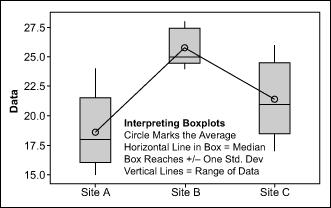Figure 2: Graphical Output – Boxplot

The graphical output from the ANOVA analysis is easy to interpret once the format being used by the statistical program is understood. The example in Figure 2 is a boxplot, typical output from statistical software.

The two key features of a boxplot are the location of the circles, denoting the mean or average for each site, and the range of the shaded gray boxes, which are drawn at plus and minus one standard deviation. Compare where the circle (average) for item falls relative to the gray boxes for the other items. If the two overlap, then they are not “statistically different.” If they do not overlap, it can be concluded that they are different.

In this case, for example, the circle (average) for Site C falls within the values marked by the gray box for Site A. So based on this data, Site A is not statistically different from Site C. However, the circle (average) for Site B does not fall within the gray-box values for either Site A or Site C, so it is significantly different from those sites.

### Acting on the Results of ANOVA

Knowing that the goal was to optimize the loan application times, what path should be taken, given these results? Odds are that there are major differences in how Site B handles the loan applications compared to Site A and Site C. At the very least, the company would want to bring Site B up to the speed of the other two sites. Thus, the first step would be to compare the loan application processes across all three sites and see how Site B differs in its policies or procedures. Once all three sites were operating the same way, then the company can look for further improvements across the board.

Handpicked Content:   How To Compare Data Sets – ANOVA

### Conclusion: Aid for Improve Phase

In Six Sigma projects, one of the biggest challenges is often whether the differences which are observed are significant enough to warrant action. One often overlooked tool that helps project teams make definitive conclusions is ANOVA. Analysis of variance is appropriate whenever continuous data from two or more groups or categories are being compared.

A better understanding of the calculations used to generate the numerical and graphical results can be found in the book Statistics for Experiments by George Box, et al. Or, those using ANOVA for the first time should be able to get help setting up the data in a statistical software program from an experienced Black Belt or Master Black Belt.

However, as shown in the example, both the numerical and graphical output from the ANOVA tests are easy to interpret. The knowledge gained will help the project team plan its improvement approach.

##### Lisa Custer

1.david

Article is generaly ok but significcant correction is needed after figure 2 where it incorrectly describes the common use of the box of a boxplot (should be interquartille range not standard deviation). Furthermore, statistical differences are shown by overlap of confidence interval of the mean, not the standard deviation (see the minitab text based graph at the bottom of figure 1).

David

2.Kicab

There is an error in the second part of the analysis: “In this case, for example, the circle (average) for Site C falls within the values marked by the gray box for Site A. So based on this data, Site A is not statistically different from Site C. However, the circle (average) for Site B does not fall within the gray-box values for either Site A or Site C, so it is significantly different from those sites.”

it’s not clear how the box plots were constructed but the default setting for box plots is that the box covers the middle 50% of the data from the 25th percentile (or 1st quartile) to the 75th percentile (or 3rd quartile). In this case, it is wrong to say that they represent +/- 1 standard deviation as stated in Figure 2–unless these were constructed specifically to be that. My software shows the same box plots so the boxes are not one standard deviation from median or mean (although it is quite close).

However, if they were, using +/- one standard deviation would not be a test at 95% confidence level and the test of means would use not the standard deviations of the data for each group but the standard error of the means, which is the data standard deviation divided by the square root of n.

More importantly, the box plots are unnecessary as the two analyses are included in the output shown in Figure 1. You correctly stated that the p value is 0.007 indicating that there is a statistical difference among two or more groups. Since we have three groups, we must then determine which groups are different.

To see which means are different you need to look at the confidence intervals shown at the bottom of Figure 1. They are the dotted lines with parentheses at each end and an asterisk in the middle, indicating the sample mean for that group. The means that do not overlap are statistically different.

Thus, we see that Site B mean is statistically different (at 95% confidence) from Site A mean but NOT statistically different from Site C mean. Site A and Site C means are also not statistically different.

Now we have a not uncommon conclusion with statistical analyses: Site B mean is not different than Site C mean which is not different than Site A mean. Doesn’t that imply that Site B mean is not different than Site A mean? No, because we are only testing whether we can see a difference not whether they are mathematically identical.

Think of it this way using colors. White is not the same as black. But we can show shades of gray from white to black such that any two consecutive shades are not visibly different to you or me. To see whether they are different we would need a more powerful “eye.” Similarly, in this case Site B mean may be actually different than Site C mean but the sample size was too small to have that powerful enough “eye.”

One minor comment: The title of the article is “When Does a Difference Matter?” I was expecting a discussion on practical significance and not on statistical significance. Perhaps the next article.

3.Dr.S.VINCENT DE PAUL

U have explained the graph very clearly, Keep this

4.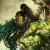Don Hutchcraft

This article showed me that to better analyze my performance, I need to run an ANOVA to see the difference in what “jobs” give am a statistical difference in how I performed the jobs. I was trying to run straight graphical charts. I may try this instead to see where the difference lies and if ti truly matters.

5.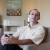Norbert Feher

Basically it is a good article. Thank You for sharing with the forum.
Anova is a great tool in case of attributive X’s and quantitative Y.

My issue is alway the very low sample size per subgroup. A sample size of 5 seems very low. As I learnt in order to be able to estimate standard deviation properly we need minimum 10 datapoints per subgroup and I always encourage green belts do do so. In case of a sample size of lower than 10 I would always look at the range.

I know it is just an example and I fully agree with the point:
Something that is significant statistically has to be significant practically and vice versa

Regards,

Norbert

6.Jud VanWyk

Statistically significant doesn’t necessarily equate to practically significant. ANOVA addresses the former; “Does it matter?” seems to imply (at least to me) the latter.

7.Thom

Nice article Lisa. This is not a simple topic. In addition to points made earlier regarding the Box Plots as well as practical and statistical differences, I would like to add:

Test for equal variances: this is one of the assumptions associated with ANOVA, and should be performed. This is where you may be concerned with the low sample sizes that Norbert indicated. There may be an interesting story with Site B: they have the highest mean, but they appear to have the least variation. This could point out one of those practical differences as well.

Multiple comparisons: this can help narrow frame the identification of practical differences. One note of caution: Minitab does return confidence intervals for the groups, but these are individual CIs. Formal multiple comparisons assists in identifying what groups actually differ based on the “family error rates.” I believe Minitab offers the ability to explore multiple comparisons

8.Drew

Kisa,
Nice article to begin the discussion of ANOVA.
In addition to the comment previously, I am not sure your definition of “six Sigma” is correct.
“(“Sigma” is the statistical notation used to represent one standard deviation; the term “Six Sigma” is used to indicated that a process is so good that six standard deviations – three above and three below the mean – fit within the specification limits)”
I believe that six sigma refers to the number of standard deviations that fit between the mean and the closest specification limit.

9.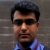Amit Kumar Ojha

Hi,

Would like to reply to Drew that Six Sigma implies six and not three standard deviations between mean and each specification limits. In case you think that there are 3 SD between mean and closest specification limits than what would happen in case there is only one specification limit(USL or LSL). In such case the process would never be six sigma if there are only 3 SD between mean and closest specification limit.